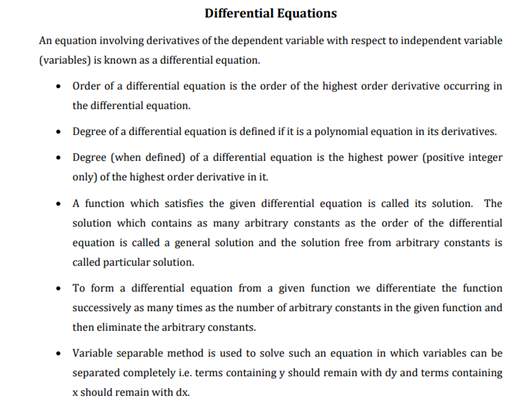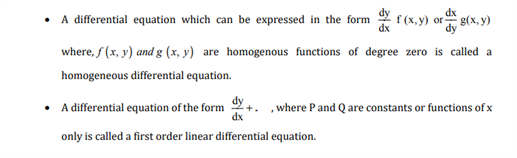# Maths Revision Notes for Class 12 Chapter 9 Differential Equations.

Chapter 9 for class maths is titled as Differential equations, which is an important chapter whose revision notes can be found below. This chapter tells students about the important concepts of differential equations. The different topics introduced in this chapter helps in making the students understand the real-life applications of differential equations. Here students can learn the definition of differential equations, which is useful in helping them understand the concepts better. Students are also taught about the order and degree of differential equations. Other important concepts that are introduced in this chapter, are the formation of ordinary differential equations and solution of a differential equation. These are important concepts that need to be learnt by students while preparing for the main exam.

The PDF for CBSE Class 12 Maths revision notes for chapter 9 Differential equations can be found below:You may also want to read:

 Derivatives Calculus
 NCERT Related Articles NCERT Solutions for Class 12 NCERT Solutions for Class 11 NCERT Solutions for Class 10 NCERT Solutions for Class 12 Maths NCERT Solutions for Class 11 Maths NCERT Solutions for Class 10 Maths NCERT Solutions for Class 12 Physics NCERT Solutions for Class 11 Physics NCERT Solutions for Class 10 Science NCERT Solutions for Class 12 Chemistry NCERT Solutions for Class 11 Chemistry NCERT Solutions for Class 6 NCERT Solutions for Class 12 Biology NCERT Solutions for Class 11 Biology NCERT Solutions for Class 6 Maths NCERT Solutions for Class 9 NCERT Solutions for Class 8 NCERT Solutions for Class 6 Science NCERT Solutions for Class 9 Maths NCERT Solutions for Class 8 Maths NCERT Solutions for Class 4 NCERT Solutions for Class 9 Science NCERT Solutions for Class 8 Science NCERT Solutions for Class 4 Maths NCERT Solutions for Class 5 NCERT Solutions for Class 7 NCERT Solutions for Class 7 Maths NCERT Solutions for Class 4 Science NCERT Solutions for Class 5 Maths NCERT Solutions for Class 7 Science NCERT Solutions NCERT Books NCERT Solutions for Class 5 Science